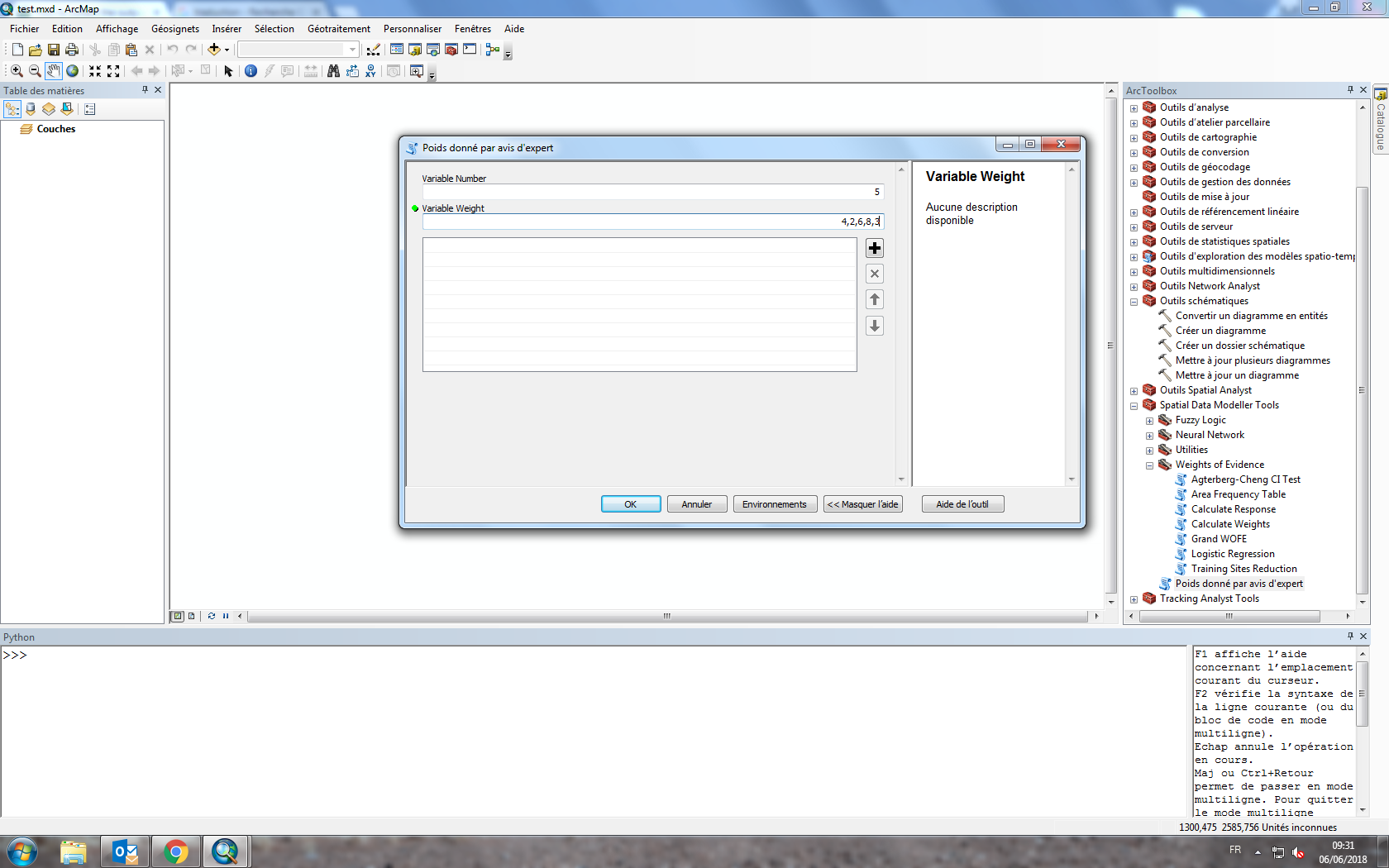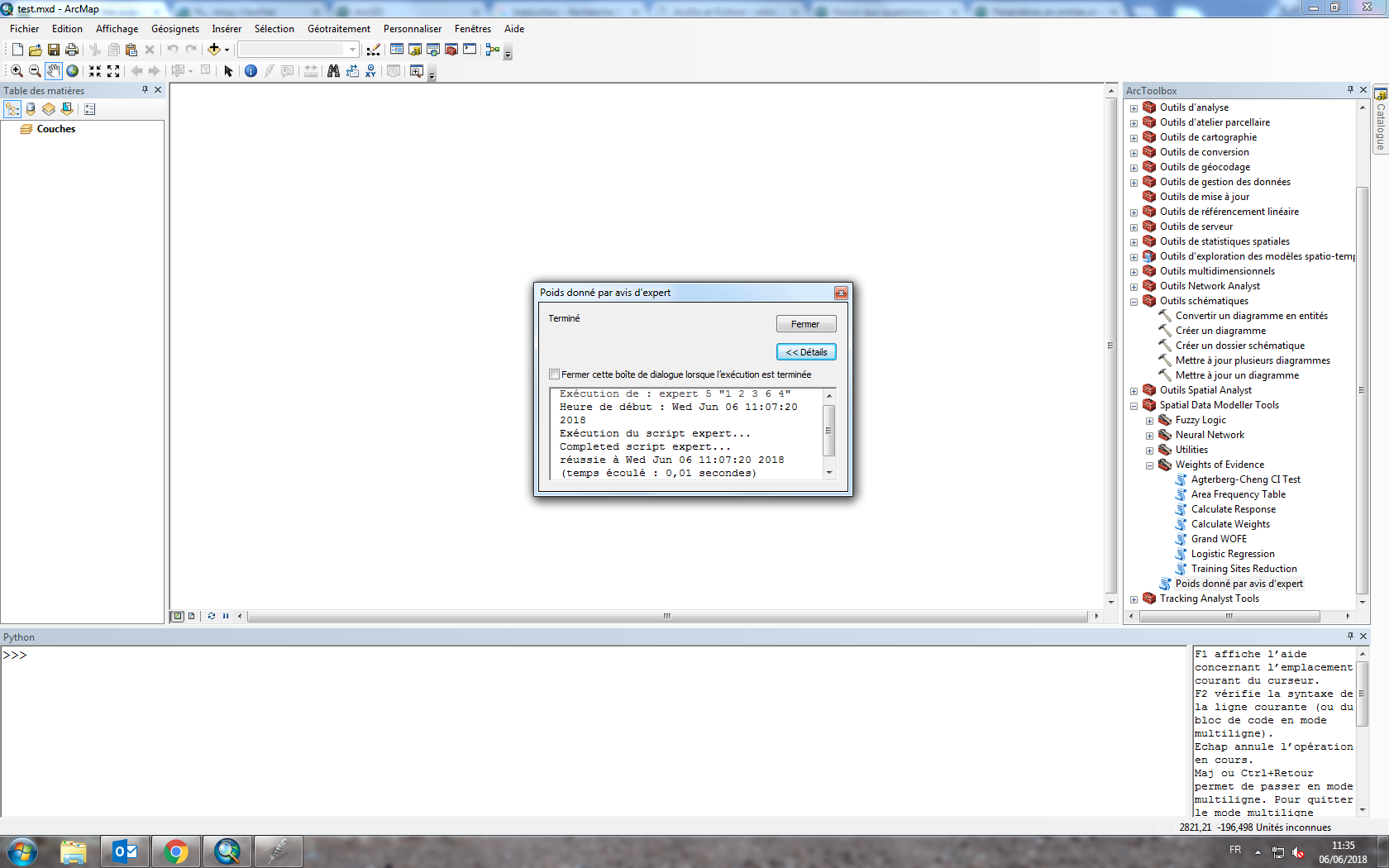# How to collect the outputs in a scripts python for arcgis ?

478
11
06-05-2018 08:22 AMNew Contributor III

Hi everybody,

I just begin to work with argis and after a long and hard work for loading the arcpy module, i face a new problem.

I'm sure it is pretty simple but i do not have all the habits yet.

I have to make a script in python, in the script the user gives the number of variables and all the weights of these variables and the script return the new weight of these variables normalised.

• Example, 2 variables that have the weight 2 and 4 and the script return 2/6 and 4/6

The code is easy, i use arcpy.GetParameter to get Var_Number but i dont know how to iterate on it, and how to return the new weight.

here is my codeTags (3)
1 Solution

Accepted SolutionsbyMVP Esteemed Contributor

Do you just want to see it? or do you intend to use it later on?

``arcpy.AddMessage(str(yourarray))‍``

will 'print' it as a result

11 RepliesbyMVP Esteemed Contributor

If you are using numpy, why are you looping?  input your 2nd variable as a list comma-separated string, then parse and convert to either integer or float.  The final array will have to be of float kind unless you want to specify the array dtypes to produce a structured or recarray.

Here is an example removing the looping

``````import numpy as np

x = np.zeros((5, 3))

x = np.zeros((5, 3))

x[:,0] = np.arange(5)  # whatever

x[:,1] = np.array([1,3,2,5,4])# whatever weights

s = np.sum(x[:,1])

x[:,2] = x[:,1]/s

x
array([[0.        , 1.        , 0.06666667],
[1.        , 3.        , 0.2       ],
[2.        , 2.        , 0.13333333],
[3.        , 5.        , 0.33333333],
[4.        , 4.        , 0.26666667]])
‍‍‍‍‍‍‍‍‍‍‍‍‍‍‍‍‍‍‍‍‍‍``````New Contributor III

In fact the code is ok,thank you for this correction, i admit i am a beginner in python.

My problem is to make this script for arcgis. The user will give first the variable number, then he gives each weight and finally argis returns a list with the new weight for example

i think the input parameters are obtained with the function getparameter, but how can i display the output on argis ?

• i have something like this• if i run iti have an error with the comma, but without i have this :I think i will have to get my output nowbyMVP Esteemed Contributor

Paul, if you are working with small arrays you could make your weight a multivalue parameter and enter the weights one at a time ( I am using pro so I don't have an example on hand.  Alternately you could specify a string parameter and parse it into its component parts.  This is demonstrated with some annotation here

``````import numpy as np

cols = 5  # your GetParameter(0)
wghts = "1 3 2 5 4"  # your GetParameter(1)  a string representation of a list
wghts = [int(i) for i in wghts.split(" ")]  # a simple list comprehension to split the text

x = np.zeros((cols, 3), dtype=np.float64)  # create the empty array
x[:, 0] = np.arange(cols)  # your index column
x[:, 1] = np.array(wghts)  # throw your weights in the 2nd column
s = np.sum(x[:,1])  # sum the weights
x[:, 2] = x[:,1]/s  # standardize relative to column 2's sum

print("demo ...\n{}".format(x))

demo ...
[[0.         1.         0.06666667]
[1.         3.         0.2       ]
[2.         2.         0.13333333]
[3.         5.         0.33333333]
[4.         4.         0.26666667]]‍‍‍‍‍‍‍‍‍‍‍‍‍‍‍‍‍‍‍‍‍‍‍‍‍‍‍‍‍‍‍‍‍‍‍‍‍‍‍‍``````

Alternately if you want a structured array (or even a recarray) you can specify the dtype for each column and assign column/field names to provide context and provide access to the data by name rather than index number...

For example

``````# ---- i4 is 32 bit integer, f8 is 64 bit float (double)
# reading from the docs assigned ;)
# note.... when the array is specified, all you do is specify the rows and not
# the number of columns... that is handled by the dtype... long story, go read my blog
# on geonet

dt = [('ID', '<i4'), ('Weight', '<f8'), ('Standard_wght', '<f8')]  # column data types
#
#create the array and assign values by column... same-ish as before
#
x = np.zeros((cols,), dtype=dt)  # create the empty array
x['ID'] = np.arange(cols)  # your index
x['Weight'] = np.array(wghts)  # throw your weights in
s = np.sum(x['Weight'])  # sum the weights
x['Standard_wght'] = x['Weight']/s
print("demo ...\n{!r:}".format(x))

demo ...
array([(0, 1., 0.06666667), (1, 3., 0.2       ), (2, 2., 0.13333333),
(3, 5., 0.33333333), (4, 4., 0.26666667)],
dtype=[('ID', '<i4'), ('Weight', '<f8'), ('Standard_wght', '<f8')])

# structured arrays don't print the exact same as uniform dtype ndarrays, but don't

# access to the data is now by column/field 'name' ie.... get the weights as an
# ndarray

x['Weight']
array([1., 3., 2., 5., 4.])

# amazing isn't it ;)‍‍‍‍‍‍‍‍‍‍‍‍‍‍‍‍‍‍‍‍‍‍‍‍‍‍‍‍‍‍‍‍‍‍‍‍‍‍‍‍‍‍‍‍‍‍‍‍‍‍‍‍‍‍‍‍‍‍‍‍‍‍‍‍‍‍``````

I have about 100 blog posts on geonet

The Py blog.... on numpy and related function and i have code on the

code sharing site search using my nameNew Contributor III

Hey Dan,

Thank you for this amazing answer, using a string parameter seems to work. I use your first code in my script.

When the add is launched everything is ok, a window opens but the output does not appear in it.

I tried to make a function and return it or to use a print but i have the same thingIs this where the ouputs should appear ?

I see your blog, i have many things to do on argis and i'm pretty sure it will be very usefull for me

I saw your page about patterns sequence occurence and positions, concerning the concave hulls, did you used a simulated annealing approach ?byEsri Esteemed Contributor

The output can be returned using  SetParameter—Help | ArcGIS DesktopbyMVP Esteemed Contributor

If you want an optional output to save to disk in the form of a *.npy file, it entails specifying a folder as the saving location and a *.npy filename which you then piece together

``````out_folder = sys.argv  # output folder name  this would be arcpy.GetParameterAsText(2) as a 'Folder' input type
out_filename = sys.argv  #arcpy.GetParameterAsText(3)  String type... output direction
out_name = "\\".join([out_folder, out_filename])‍‍‍``````

ie c:/temp/array.npy

From there you can bring it back into numpy using

``np.load("c:/temp/array.npy")‍``New Contributor III

My output is in the third position in my parameter list so i tried something like this. However i still not have the final array.

import numpy as np
import arcpy

lines = arcpy.GetParameter(0)                                                       # number of lines of the array (variables)
wghts = arcpy.GetParameter(1)
wghts = [int(i) for i in wghts.split(" ")]                                              # weight of these variables

x = np.zeros((lines, 3))
x[:, 0] = np.arange(1,lines+1)                                                         # we number the variables in this column
x[:, 1] = np.array(wghts)                                                                 # the weight in this one
s = np.sum(x[:,1])
x[:, 2] = x[:,1]/float(s)                                                                      # the normalized weight in this one

arcpy.SetParameter(2, x)                                                               # arcgis type : long integer and multiple value

Sorry for annoying you haha this is the first time i use arcgisbyMVP Esteemed Contributor

Do you just want to see it? or do you intend to use it later on?

``arcpy.AddMessage(str(yourarray))‍``

will 'print' it as a resultbyMVP Esteemed Contributor

Your result won't be multivalue nor will it be integer

You will need to convert it to a string

ie  str(x)